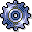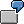FormulasUse

A formula is a logical or mathematical expression used to calculate numerical values, such as a + b. You can use basic expressions to construct formulas of any degree of complexity required. In addition, you can use functions from the currently valid environment, or you can use constant values.

Allowed Arithmetic Operators

The following is allowed: Terms from the provided functions or figures (including decimal figures) that are connected by the four arithmetic operators (+,-,*,/). These can, in turn, be separated from each other by parentheses. In addition, special mathematics functions can be used.1. Simple: Function

2. (Function1 + 2) * (4 - Function2)

3. Mathematical function: Ceil (Function1 * Function2) / Floor (Function3 * 0,5)

The following is not allowed:

• The comparison operators: = <> <= >= <>
• The Boolean operators: AND OR
• The quantity operator: IN